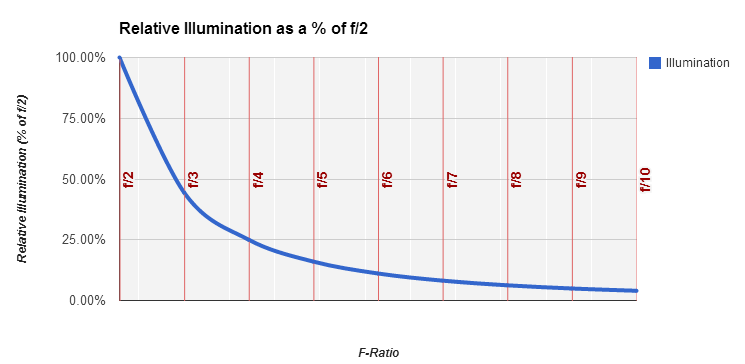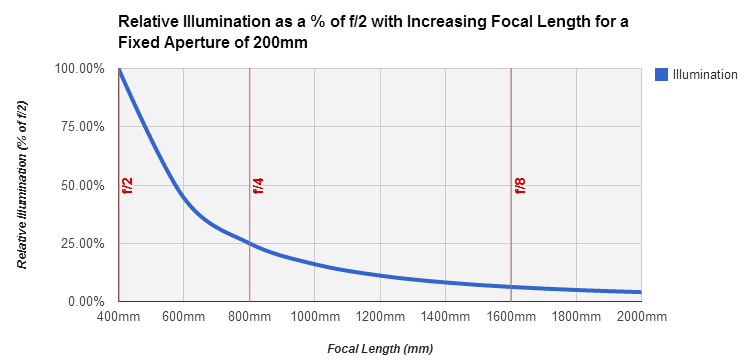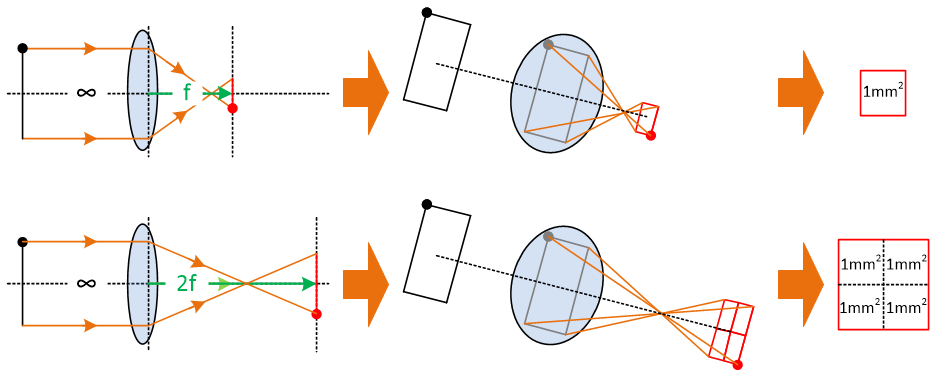## Thursday, 16 October 2014

### Basics Part 1: Understanding Aperture, Focal Length and Focal RatiosQuestions about the best choice of equipment for astro-imaging come up quite frequently. In this first of a series of posts, I will start by explaining the basics of aperture, focal length focal ratios.

These troublesome topics cause many beginning imagers to jump to mistaken conclusions. If you bear with me you'll find out how they (and other things) affect imaging and consequently you will be able to make better informed choices about your equipment. Read on for more...

### So what is a focal ratio?

"Focal ratio" (f-ratio) is a common term you will come across in the world of photography. It is a number that is used as shorthand for the "speed" of a camera lens and is expressed as "f/2", "f/4", etc. Lower numbers are "faster" than higher numbers, meaning that you can take a photograph using a shorter exposure length with an f/2 lens than you can with an f/4 lens. The simple rules used in photography don't carry straight over to astro-imaging though, as we'll find out.

### Calculating f-ratios

The f-ratio of a camera lens or a telescope can be calculated very simply as:

f-ratio = focal length / aperture

So for example, my old Meade SCT has a focal length of 2,000mm and it has an aperture of 203mm (i.e. the mirror has a diameter of 203mm). Therefore its f-ratio is 2,000 / 203 = f/9.85. It is fairly common to round higher f-ratios up or down, so this scope was advertised as having a f-ratio of f/10.

To give another example, my SkyWatcher 80ED has a focal length of 600mm and an aperture of 80mm and thus it is an f/7.5.

If you are using a focal reducer or a Barlow lens in your set-up, you should include the resulting decrease or increase in effective focal length in the calculation as follows:

f-ratio = focal length x change factor / aperture

So if I use my 0.85x focal reducer with my 80ED, the calculation becomes 600mm x 0.85 (510mm effective focal length) with an aperture of 80mm equals f/6.375 so let's call it f/6.4.

Using a 2x Barlow the effective focal length becomes 600mm x 2 which is 1,200mm making for f/15.

Note: The f-ratio is a dimensionless number, i.e. it does not have any units. Provided you use the same units for both aperture and focal length you can calculate the f-ratio correctly. Don't mix up mm and inches in one calculation!

### A better definition of f-ratio

Unfortunately that shorthand of equating f-ratios to speed or exposure time has the potential to be very misleading when it comes to astro-imaging. So let's use a more precise definition:

The f-ratio determines how much light a telescope (or lens) will concentrate in to a given area.

So if I point my two telescopes at uniform source of light such as an overcast sky, the one with the lower f-ratio will illuminate a given area of the camera sensor more than the one with the higher f-ratio.

Now you may think I'm just nit-picking here. Surely if a lower f-ratio illuminates the sensor more, then any object you are trying to image will appear brighter to your camera and thus you will be able to take a shorter exposure? Yes and no; the image will appear brighter but that doesn't necessarily mean you can take a shorter exposure. Bear with me a whilst we explore f-ratios a bit further and we'll come back to that point later in the series...

### F-ratios and illumination

Firstly, let's explore how much light you get for each f-ratio. The graph below shows how much less light would fall on a given area (say a pixel in your camera sensor) as you increase the f-ratio. I've arbitrarily chosen f/2 as the staring point and called that 100%, and then shown what percentage of light would fall in the same area on the camera sensor as we increase the f-ratio.Relative illumination with increasing f-ratios
The simple rule is that every time you double the f-ratio, you get a quarter of the light of the previous ratio.

So for f/4 we get 25% of the light of f/2 in the same area, and for f/8 we get 25% of the light of f/4 and thus 6.25% of the light of f/2 (a quarter of a quarter).

Bear in mind that I arbitrarily started at f/2, but the relationship applies to any series of f-ratios. So f/10 would give you a quarter of the illumination of f/5, and f/6 would give you a quarter of the illumination of f/3.

### F-ratios and aperture

There are two ways in which we can vary the f-ratio of our telescope (or lens). One way is to change the f-ratio by changing the aperture and keeping the focal length the same. I've illustrated this below:Relative illumination with decreasing aperture
So let's say we have a telescope with a focal length of 800mm. If we have an aperture of 400mm, then it is an f/2 scope, but if we reduce the aperture to 200mm it becomes an f/4 scope, and if we further reduce the aperture to 100mm then it becomes an f/8.

In terms of illumination, the relationship is the same as we explored for f-ratios above - halve the aperture (whilst keeping the same focal length) and you will get a quarter of of the illumination. There is no magic involved here, it's a simple matter of geometry.

By halving the aperture of the objective, its light-gathering area is reduced to a quarter of the original. One quarter of the light coming in to the front of the scope equals one quarter of the light coming out of the back equals one quarter of the illumination (assuming we keep the same focal length).

For example, an aperture of 200mm has an area of 31,416mm(pi x radius2). An aperture of 100mm aperture covers 7,854mm2. 7,854 / 31,416 = 0.25 (i.e. one quarter of the area).

Note: Telescopes have fixed apertures, so if you want a bigger aperture you have to buy a bigger scope. Camera lenses have a Diaphragm which allow the aperture to be made smaller or larger as required. If you are a photographer looking for your first telescope, it is easy to fall in to the trap of assuming that a faster f-ratio always equals more light when in fact it might be due to focal length...

### F-ratios and focal length

The second option for changing the f-ratio is to change the focal length whilst keeping the aperture constant. In terms of illumination, the following occurs:Relative illumination with increasing focal length
So if we have a fixed aperture of 200mm and a focal length of 400mm, we have an f/2 system. Increasing the focal length to 800mm gives us f/4

In this case the relationship is that if you double the focal length, you get a quarter of the illumination. Again, it's a simple matter of geometry as you can see from the illustration below:Effect of increasing focal length
We're imaging objects that are at infinity, so doubling the focal length of your scope or lens will also double the "magnification". An image of a straight line line that is 1mm long on the the camera sensor at a focal length of 500mm would be 2mm long if you increased the focal length to 1,000mm (left hand side of the illustration above).

But we are concerned about how much light falls in an area. So if 100% of the incoming light falls in a box of 1mm x 1mm = 1mm2 at a focal length of 500mm, then it will fall in a box of 2mm x 2mm = 4mm2 at a focal length of 1,000mm (middle of the illustration).

So at 1,000mm focal length we have the same amount of light falling in 4 x 1mm2 boxes, which means that a quarter of the light falls in each of the 1mm2 boxes as fell in the original 1mm2 box at 500mm focal length (right hand side of the illustration).

In the real world the boxes that we are concerned about are the pixels on our camera sensor. By doubling the focal length, we put one quarter as much of the incoming light on each pixel.

Note 1: We aren't really imaging objects that are infinitely far away, but they are as close to infinity as makes no practical difference to the optics of your telescope or lens.

Note 2: Fitting an image of a galaxy that is hundreds of thousands of light years across on to a sensor that is 20 or 30mm across hardly counts as magnification! When we use the term "magnification" in this context, we should really say that we are increasing the apparent angular separation of features in the image.

There is an important rider on focal length, in that it breaks down when we start dealing with Point Sources. A point source is anything which:

- Has an angular size that too small to be Resolved by your telescope; stars most certainly fit this definition.

- Is resolved by your telescope but not by your camera due to large sensor pixels or a short focal length, i.e. the light from the object falls entirely on to a single camera pixel. (We'll come back to this in Part 2).

In the case of a point source, focal length has no relevance; the brightness of the object at the camera sensor is determined entirely by the aperture of the scope. In other words, you can't make it any brighter by reducing the focal length (thus reducing the "magnification") - it's already as small as it can be.

### All f-ratios are not created equal

The observant amongst you will have noticed that the two methods of changing f-ratio have very different consequences. Increasing aperture leads to a lower ("faster") f-ratio by gathering more light, whereas reducing focal length does the same by shrinking the image and thus concentrating the same amount of light on to a smaller area.

So a 200mm aperture / 2,000mm focal length telescope and a 100mm aperture / 1,000mm focal length telescope are both f/10. The former will gather four times as many photons as the latter in the same amount of time, but it will also spread them out over an area that is four times as large. If we use the same camera on both telescopes, the sensor pixels will receive the same number of photons in each case.

So that means one f/10 scope is just as good as another, right? Not necessarily:

- Firstly, the shorter focal length instrument will project a half-sized image on to your camera sensor, and thus the resulting image of the target will consist of a quarter as many pixels. Whether this matters or not depends on the size of your camera pixels.

- Secondly, the quality of your image may be better or worse depending on the brightness of your target, the brightness of the sky and the amount of read noise produced by your camera.

We'll examine these issues in more detail in Part 2 of this series before drawing some conclusions.